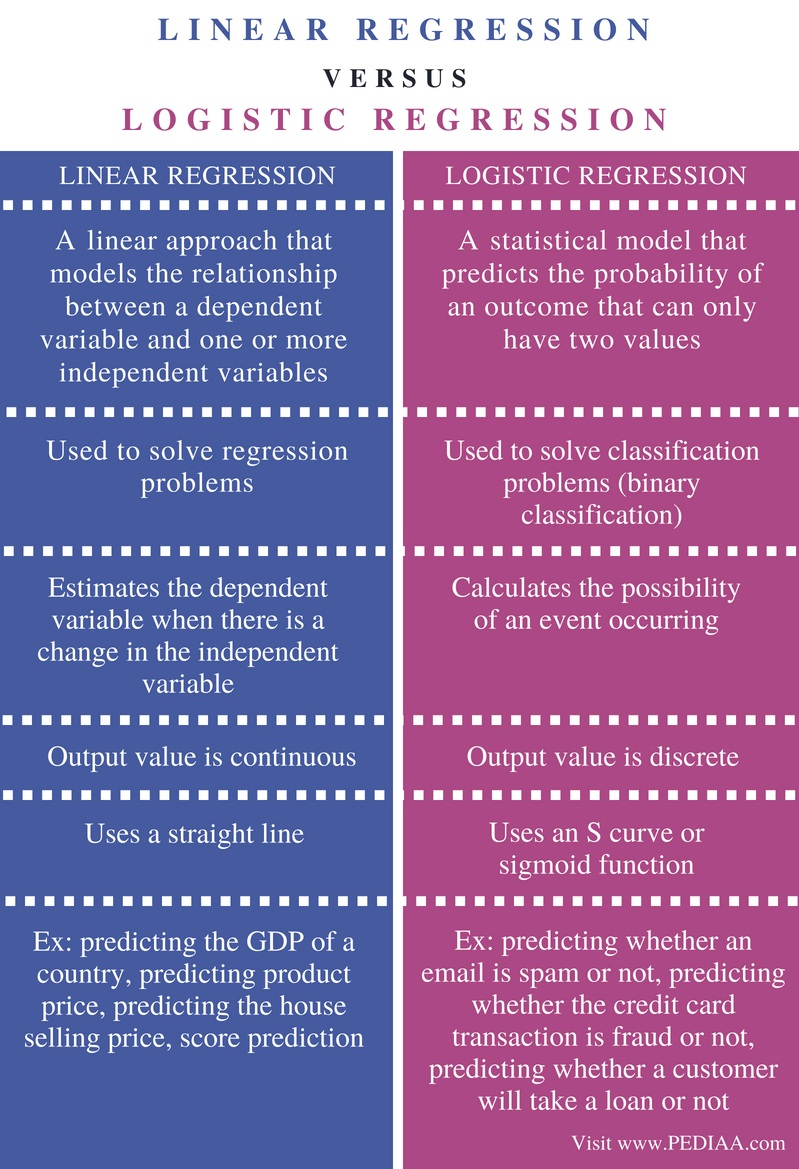# Difference Between Linear Regression and Logistic Regression

The main difference between linear regression and logistic regression is that the linear regression is used to predict a continuous value while the logistic regression is used to predict a discrete value.

Machine learning systems can predict future outcomes based on training of past inputs. There are two major types of machine learning called supervised learning and unsupervised learning. Regression and classification fall under supervised learning while clustering falls under unsupervised learning. Supervised learning algorithms use labeled data to train the data set. Linear regression and logistic regression are two types of supervised learning algorithms. Linear regression is used when the dependent variable is continuous, and the model is linear. Logistic regression is used when the dependent variable is discrete, and the model is nonlinear.

### Key Areas Covered

1. What is Linear Regression
– Definition, Functionality
2. What is Logistic Regression
– Definition, Functionality
3. Difference Between Linear Regression and Logistic Regression
– Comparison of Key Differences

### Key Terms

Linear Regression, Logistic Regression, Machine Learning## What is Linear Regression

Linear regression finds the relationship between independent and dependent variables. Both of them are contiguous. The independent variable is the variable that is not changed by the other variables. It is denoted by x. There can also be multiple independent variables such as x1, x2, x3, etc. Dependent variable changes according to the independent variable, and is denoted by y.

When there is one independent variable, the regression equation is as follows.

y = b0+ b1x

For example, assume that x represents rainfall and y represents the crop yield.Figure 1: Linear Regression

The dataset will look like above. Then, a line that covers most of the data points is selected. This line represents the predicted values.Figure 2: Distance between the actual data points and the predicted values

Then, the distance from each data point to the line is found as shown in the above graph. This is the distance between the actual value and the predicted value. This distance is also known as the error or residuals. The best fit line should have the least sum of squares of errors. When new rainfall value is given (x), it is possible to find the corresponding crop yield (y) using this line.

In the real world, there can be multiple independent variables (x1, x2, x3…).  This is called multiple linear regression. The multiple linear regression equation is as follows.

## What is Logistic Regression

Logistic regression can be used to classify two classes. It is also known as binary classification.  Checking whether an email is spam or not predicting whether a customer will buy a product or not, predicting whether it is possible to get a promotion or not are some other examples of logistic regression.Figure 3: Logistic Regression

Assume that the number of hours a student studied per day is the independent variable. Depending on that, the probability of passing an exam is calculated. The value 0.5 considered as the threshold. When the new number of hours is given, it is possible to find the corresponding probability of passing the exam using this graph. If the probability is above 0.5, it is considered as 1 or pass. If the probability is below 0.5, then it is considered as 0 or fail.

Applying the linear regression equation to the sigmoid function will give the logistic regression equation.

The sigmoid function isAnother important point to note is that logistic regression is only applicable to classify 2 classes. It is not used for multiclass classification.

## Difference Between Linear Regression and Logistic Regression

### Definition

Linear regression is a linear approach that models the relationship between a dependent variable and one or more independent variables. In contrast, logistic regression is a statistical model that predicts the probability of an outcome that can only have two values.

### Usage

While linear regression is used to solve regression problems, logistic regression is used to solve classification problems (binary classification).

### Methodology

Linear regression estimates the dependent variable when there is a change in the independent variable. Logistic regression calculates the possibility of an event occurring. This is one important difference between linear regression and logistic regression.

### Output Value

Also, in linear regression, the output value is continuous. In logistic regression, the output value is discrete.

### Model

Although linear regression uses a straight line, logistic regression uses an S curve or sigmoid function.This is another important difference between linear regression and logistic regression.

### Examples

Predicting the GDP of a country, predicting product price, predicting the house selling price, score prediction are some examples of linear regression. Predicting whether an email is spam or not, predicting whether the credit card transaction is fraud or not, predicting whether a customer will take a loan or not are some examples of logistic regression.

### Conclusion

The difference between linear regression and logistic regression is that linear regression is used to predict a continuous value while logistic regression is used to predict a discrete value.  In brief, linear regression is used for regression while logistic regression is used for classification.

##### Reference:

1. Linear Regression Analysis | Linear Regression in Python | Machine Learning Algorithms | Simplilearn, 26 Mar. 2018, Available here.
2. Logistic Regression | Logistic Regression in Python | Machine Learning Algorithms | Simplilearn, 22 Mar. 2018, Available here.

##### Image Courtesy:

1. “Linear regression” By Sewaqu – Own work, Public Domain) via Commons Wikimedia
2. “Residuals for Linear Regression Fit” By Thomas.haslwanter – Own work (CC BY-SA 3.0) via Commons Wikimedia
3. “Logistic-curve” By Qef (talk) – Created from scratch with gnuplot (Public Domain) via Commons Wikimedia